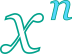## N-th power calculator

This tool evaluates the n-th power of a number: . Enter the the power degree n and the argument x below. Non integer n is supported.

 n = x = Result: x^n =## Definitions

### General

The n-th power of a number x, when n is an integer, is the result of multiplying x to itself n times (x*x*x...*x). In modern notation n-th root is written as xn. Typically degree n is an integer. For fractional powers, the result can be decomposed to an integer power and a radical. For example:

For n=2 the power is called square while for n=3 cube. For n=0 the result is always 1, while for n=1 the power result renders the same x number.

### Properties

The derivative of the principal n-th power function is:

The integral of the n-th power function is given by: